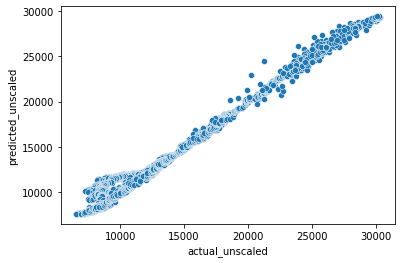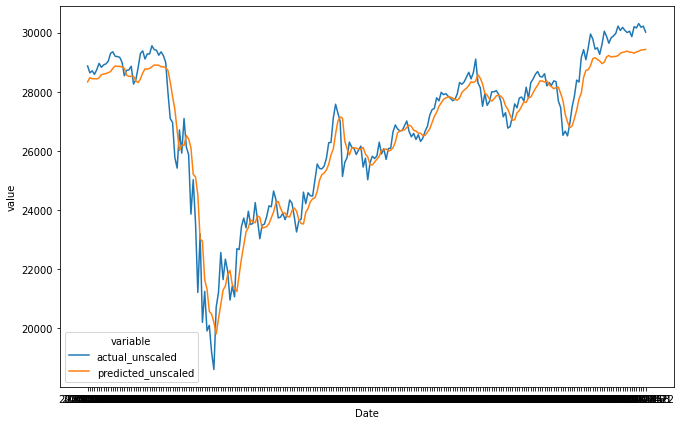Learn data science and machine learning by building real-world projects on Jovian

### Using LSTM to predict Dow Jones price

This was a great class and really got me thinking about how to apply deep learning solutions.
One of the things I'm interested in is analysis and predictions on time-series data
I did some reading and practicing outside of the course, and learned something about LSTM architecture. LSTM is a type of recurrent neural net that allows information contained in seqences of data to be leveraged in the model. In other words, relationships between successive data points can be used in the model, not just individual data points.
I also learned how to get started with the Skorch library, which is a wrapper around PyTorch that allows PyTorch models to be used in a typical Scikit-Learn pipeline. Since I use Scikit at work, I thought might be a good idea to get up to speed with Skorch.
So my general approach was to use:

• Skorch
• LSTM

To predict a time series.

I'm joining two daily datasets:

• Dow Jones Industrial Average
• Foreign exchange rates (Euro, Japanese Yen, Mexican Peso, Chines Yuan) in terms of US Dollar

And trying to use the previous 5 days (i.e. a moving window) of data to predict the closing Dow Jones price the following day

In :
``````import pandas as pd
import numpy as np
import sqlite3

from sklearn.preprocessing import StandardScaler

import torch
import torch.nn as nn
import torch.functional as F

from skorch import NeuralNetRegressor

import seaborn as sns
import matplotlib.pyplot as plt

import pickle``````
In :
``con = sqlite3.connect(":memory:")``
In :
``````#read in stock market data:
df_djia.to_sql("djia", con, if_exists="replace")``````
``` Open High Low Close \ Date 2000-01-03 11501.849609 11522.009766 11305.690430 11357.509766 2000-01-04 11349.750000 11350.059570 10986.450195 10997.929688 2000-01-05 10989.370117 11215.099609 10938.669922 11122.650391 2000-01-06 11113.370117 11313.450195 11098.450195 11253.259766 2000-01-07 11247.059570 11528.139648 11239.919922 11522.559570 Adj Close Volume Date 2000-01-03 11357.509766 169750000 2000-01-04 10997.929688 178420000 2000-01-05 11122.650391 203190000 2000-01-06 11253.259766 176550000 2000-01-07 11522.559570 184900000 ```
```/home/jeff/.local/lib/python3.8/site-packages/pandas/core/generic.py:2602: UserWarning: The spaces in these column names will not be changed. In pandas versions < 0.14, spaces were converted to underscores. sql.to_sql( ```
In :
``````#read in forex data:
df = pd.read_csv("./FRB_H10.csv", na_values=['ND'], skiprows=5, index_col="Time Period").dropna() #first 5 rows are metadata
df['EUR'] = 1/df['EUR'] #the EUR column is originally in \$/EUR, need to change to EUR/\$
df.to_sql("forex",con,if_exists="replace")``````
``` EUR CAD CNY MXN Time Period 2000-01-03 0.984737 1.4465 8.2798 9.4015 2000-01-04 0.970026 1.4518 8.2799 9.4570 2000-01-05 0.967586 1.4518 8.2798 9.5350 2000-01-06 0.968617 1.4571 8.2797 9.5670 2000-01-07 0.971440 1.4505 8.2794 9.5200 ```
```/home/jeff/.local/lib/python3.8/site-packages/pandas/core/generic.py:2602: UserWarning: The spaces in these column names will not be changed. In pandas versions < 0.14, spaces were converted to underscores. sql.to_sql( ```
In :
``````#combine the two dataframes:
df = pd.read_sql('select f.*,d.Open,d.Close from forex as f inner join djia as d on d.Date=f."Time Period"', con, index_col="Time Period")
``````
``` EUR CAD CNY MXN Open Close Time Period 2000-01-03 0.984737 1.4465 8.2798 9.4015 11501.849609 11357.509766 2000-01-04 0.970026 1.4518 8.2799 9.4570 11349.750000 10997.929688 2000-01-05 0.967586 1.4518 8.2798 9.5350 10989.370117 11122.650391 2000-01-06 0.968617 1.4571 8.2797 9.5670 11113.370117 11253.259766 2000-01-07 0.971440 1.4505 8.2794 9.5200 11247.059570 11522.559570 ```
In :
``````#check into replacing with an imputer using last value if this is a common occurrence:
df.dropna(inplace=True)``````
In :
``````#this is the scikit learn scaler, which is used to normalize data. Useful when features are of different magnitudes.
ss = StandardScaler()
``````
In :
``len(df.index.to_list())``
Out:
``5232``
In :
``````data = ss.fit_transform(df)
dates = df.index.to_list()

window_length = 5
x_list = []
y_list = []
y_list_binary = []
date_list = []

while data.shape >= (window_length + 1):
x_list.append(data[:window_length,:])
y_list.append(data[window_length, -1])
y_list_binary.append(data[window_length,-1]>data[window_length-1,-1])
date_list.append(dates[window_length])

data = data[1:,:]
dates = dates[1:]

x = torch.tensor(np.array(x_list).astype("float32"))
y = torch.tensor(np.array(y_list).reshape(-1,1).astype("float32"))
y_bin = torch.tensor(np.array(y_list_binary).reshape(-1,1).astype("float32"))
print(data[:11,:])``````
```[[-0.19458599 0.22257106 -0.81377447 1.71419948 2.70261678 2.69568583] [-0.23938483 0.20029015 -0.81402331 1.67053585 2.70689059 2.72152086] [-0.22756964 0.23426854 -0.80481621 1.72263572 2.72394822 2.69994076] [-0.22433993 0.26768991 -0.79262303 1.73845705 2.69699555 2.70643266] [-0.19295532 0.31336578 -0.80095918 1.74223045 2.70414762 2.67155209]] ```
In :
``````print(x.shape)
print(y.shape)
``````
```torch.Size([5227, 5, 6]) torch.Size([5227, 1]) ```
In :
``````#let's see if we can get output from one LSTM layer:
lstm = nn.LSTM(x.shape,16)

test_input = x.permute(1,0,2)[:,:10,:]
test_output = lstm(test_input)

print(test_input.shape)
print(test_output.shape)
#what I want is the last output for each input:
test_output[:,-1,:]``````
```torch.Size([5, 10, 6]) torch.Size([5, 10, 16]) ```
Out:
``````tensor([[ 0.0803, -0.0544, -0.0503, -0.0457, -0.1401,  0.1291, -0.1386,  0.1902,
-0.0904, -0.0334,  0.0486, -0.1708, -0.0785,  0.0066, -0.0770, -0.1144],
[ 0.1201, -0.0491, -0.0931, -0.0700, -0.1875,  0.1688, -0.1879,  0.2681,
-0.1384, -0.0297,  0.0703, -0.2514, -0.1314,  0.0549, -0.1432, -0.1720],
[ 0.1401, -0.0390, -0.1205, -0.0826, -0.2002,  0.1826, -0.2042,  0.2994,
-0.1617, -0.0171,  0.0790, -0.2822, -0.1661,  0.1089, -0.1874, -0.2018],
[ 0.1476, -0.0287, -0.1361, -0.0862, -0.2025,  0.1886, -0.2073,  0.3091,
-0.1735, -0.0087,  0.0821, -0.2930, -0.1882,  0.1540, -0.2136, -0.2171],
[ 0.1512, -0.0230, -0.1465, -0.0852, -0.2007,  0.1912, -0.2058,  0.3119,
-0.1825, -0.0017,  0.0830, -0.2947, -0.2024,  0.1867, -0.2283, -0.2223]],
In :
``````class Model(nn.Module):
def __init__(self):
super(Model, self).__init__()
self.lstm1 = nn.LSTM(x.shape,32)
self.linear1 = nn.Linear(32,16)
self.linear2 = nn.Linear(16,1)
def forward(self, x):
x = x.permute(1,0,2)
x = torch.relu(self.lstm1(x)[-1,:,:])
x = torch.relu(self.linear1(x))
x = self.linear2(x)
return x``````
In :
``````#cool, looks like the model works.
#let's use in Skorch...
model = Model()
``````
In :
``net = NeuralNetRegressor(model, max_epochs=100, device='cpu', iterator_train__shuffle=True, lr=0.01, verbose=0)``
In :
``history = net.fit(x,y)``
In :
``````#create a data frame of actual and predicted values, have columns for scaled and unscaled versions

df_predictions = pd.DataFrame({'actual':y.reshape(-1).tolist(),'predicted':model(x).reshape(-1).tolist()},index=date_list)

s = ss.scale_[-1]
m = ss.mean_[-1]

for col in df_predictions.columns:
df_predictions[f"{col}_unscaled"] = df_predictions[col]*s+m

df_predictions.index.name="Date"``````
In :
``df_predictions.to_excel("./df_predictions_forex.xlsx")``
In :
``````#here's what the predictions look like:
df_predictions.tail(10)``````
Out:
In :
``````#plot actual vs. predicted values:
sns.scatterplot(data=df_predictions,x="actual_unscaled",y="predicted_unscaled")``````
Out:
``<AxesSubplot:xlabel='actual_unscaled', ylabel='predicted_unscaled'>``In :
``````#here's what the time series of predictions vs. actual looks like
df_predictions_melted = df_predictions[["actual_unscaled","predicted_unscaled"]].reset_index().melt(id_vars=["Date"])
print(df_predictions_melted.tail())
``````
``` Date variable value 10449 2020-12-16 predicted_unscaled 29341.555403 10450 2020-12-17 predicted_unscaled 29369.685614 10451 2020-12-18 predicted_unscaled 29406.971432 10452 2020-12-21 predicted_unscaled 29419.503049 10453 2020-12-22 predicted_unscaled 29428.250733 ```
In :
``````fig, scatter = plt.subplots(figsize = (11,7))
sns.lineplot(data=df_predictions_melted.loc[df_predictions_melted["Date"]>'2020-01-01'], x="Date",y="value",hue="variable")``````
Out:
``<AxesSubplot:xlabel='Date', ylabel='value'>``### Conclusion

Although I learned a lot about using PyTorch to implement deep-learning models, I am not super happy with the results of my LSTM.
Mainly because the predictions appear to be lagging the actual by a couple of days. This could likely be because the predictions are not able to predict an upswing until the upswing is actually seen in the actual data.
I tried a bunch of different hyperparameters:

• Hidden layer size
• training epochs

But performance doesn't seem to improve after 16 cells in the hidden layer or after 100 epochs of training.

No matter what, I continued to get predictions that lag the actual. Ideally I would get predictions that follow the actual very closely. Reasons for this are:

• This is just an impossible problem to solve
• I need more data
• I need a better model

If I had more time I would like to provide more years of data and see how the model improves

In [ ]:
`` ``